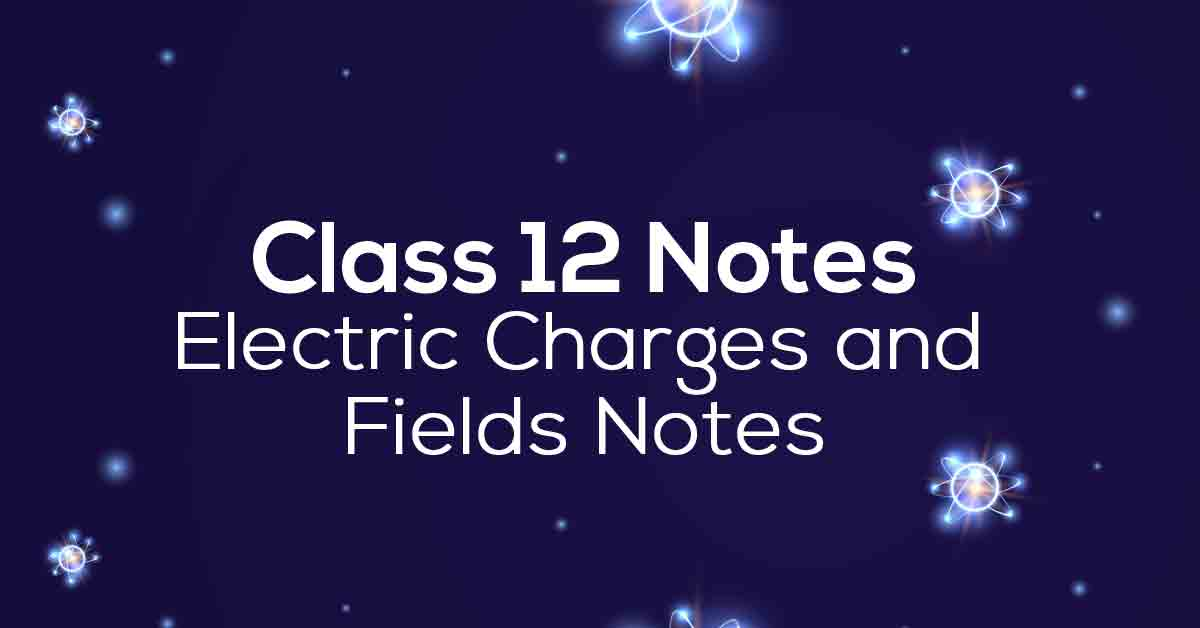Class 12th Physics Electric Charges and Fields NCERT Notes CBSE 2023

# Electric Charges and Fields Class 12 Notes# Chapter 1 Electric Charges and Fields

One of the most important topics for students to prepare for the Class 12 CBSE exam is Physics. However, the topic of each chapter must be understood by students if they want to do well on the board exams. NCERT Grade 12 notes can help students prepare for CBSE Year 12 and competitive exams like JEE and NEET. Therefore, students need to focus on solving as many problems as possible to master chapter 1 of Physics.

CBSE Grade 12 Physics Chapter 1 is about conductors and insulators, induction, Coulomb's law, electric fields, and Gauss' law. Besides that, it also talks about electric dipole, current, continuous charge distribution, and much more. Vidyakul provides a set of practice questions for all the sub-topics included in the chapter for you to practice. In addition, students should emphasize rehearsing questions in Vidyakul's writing to score maximum marks. This article provides NCERT notes for Grade 12 Physics Chapter 1 and key topics.

### Points to Remember

• Coulomb’s law states that the force of attraction or repulsion between two charged bodies is inversely proportional to the square of the distance between them. It is directly proportional to the product of their charges.

• There are two types of charges in nature; positive charge and negative charge. Like charges repel each other and unlike charges attract each other.

• In conductors, electric current flows due to the movement of electrons through them, in insulators electric current cannot flow.

• The region around a charge in which its electrical effects can be observed is called the electric field of that charge.

• An electric field line is a curve drawn in such a way that the tangent at each point on the curve gives the direction of the electric field at that point.

• Two field lines cannot cross each other.

• Electrostatic field lines start at positive charges. They end at negative charges.

### Topics and Sub-topics

Below, we have provided the list of topics included in this chapter:

 Exercise Topic Description 1 Electric Charge 2 Conductors, Insulators and Semiconductors 3 Different Methods of Charging 4 Basic Properties of Electric Charge 5 Coulomb’s Law 6 Superposition Principle 7 Electric Field 8 Electric Field Lines 9 Electric Flux 10 Electric Dipole 11 Dipole in a Uniform External Field 12 Continuous Charge Distribution

Download the FREE PDF of Electric Charges and Fields Class 12 Notes and start your preparation with Vidyakul!

Download Vidyakul App for more Important notes, PDF's and Free video lectures.

### Few Important Questions

• What is meant by ‘Induction’?

Induction is the property of a conductor to become electrified when there is the presence of a charged body present nearby.

• What does Coulomb’s Law state?

Coulomb’s Law: The force of attraction or repulsion acting along a straight line between two electric charges is directly proportional to the product of the charges and inversely to the square of the distance between them.

• What is ‘electric flux’?

The measure of the count of the number of electric field lines crossing an area is known as Electric Flux.

### Practice Questions

1. (a) The Statement needs an explanation ‘electric charge of a body is quantized’.

(b) Why can one ignore the quantization of electric charge when dealing with macroscopic i.e., large-scale charges?

2. Explain how the law of conservation of charge works on this phenomenon when charges appear on both the silk cloth and the glass rod when they are rubbed together.?

(a)Why electrostatic field line is not a continuous curve and cannot have sudden breaks?

(b) Why do two field lines never cross each other at any point?